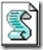# VBScript vs PowerShellI am converting an old VBScript to PowerShell and it’s very nice to see how many lines of code in VBS can be written in one line of (much better readable) code in PowerShell.

Take this example VBS code that creates a formatted Date Time string:

```' Datum en tijd variabelen
dD = Day(Date)
dM = Month(Date)
dY = Year(Date)
tH = Hour(Now)
tM = Minute(Now)
tS = Second(Now)

' Alles onder de waarde tien wordt het cijfer "0" voorgezet.
If dD < 10 Then dD = "0" & dD
If dM < 10 Then dM = "0" & dM
If tM < 10 Then tM = "0" & tM
If tH < 10 Then tH = "0" & tH
If tS < 10 Then tS = "0" & tS

' datum en tijd notatie wordt opgeslagen in deze variabel
CRDate = dD & "\" & dM & "\" & dY & " - " & tH & ":" & tM & ":" & tS```

And in PowerShell:

`\$CrDate = "{0:dd\\MM\\yy - HH:mm:ss}" -f (Get-Date)`

EDIT: thanks to @ShayLevy for pointing out that Get-Date has a format operator:

```Get-Date -format 'dd\\MM\\yy – HH:mm:ss'
```
Tags: